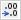# How to Round a number in Excel?

• Applies to: Excel for Microsoft 365, Excel for the web, Excel 2019, Excel 2016, Excel 2013, Excel 2010, Excel 2007.

In this post, you will learn how to round a number in Excel. We will cover the following:

• Firstly, how to adjust the number of decimal places presented without actually altering the number;
• Secondly, how to round a number up;
• Thirdly, how to round a number down;
• Then, how to round a number to the nearest number;
• Next, how to round a number to a near fraction;
• After this, how to round a number to a significant digit; and
• Finally, how to round a number to a specified multiple.

Imagine that you seek to round a number to the nearest whole number since decimal values are of little importance to you. Alternatively, you want to round a number to multiples of 10 to make an estimation of approximation of amounts easier. There are multiple methods for how to round a number in Excel.Image by Free-Photos from Pixabay.

## Change the number of decimal places displayed without changing the number

### On a worksheet

1. Click the cells that you are looking to format.
2. To show more or fewer digits after the decimal point, do the following. On the Home tab, in the Number group, select Increase Decimalor Decrease Decimal.

### In a built-in number format

1. On the Home tab, in the Number group, press the arrow beside the list of number formats, and then choose More Number Formats.2. In the Category list, according to the data type of your numbers, press CurrencyAccountingPercentage, or Scientific.
3. In the Decimal places box, type the amount of decimal places that you prefer to present.

## Round a number up

Employ the ROUNDUP function. For some scenarios, you might prefer to apply the EVEN and the ODD functions to round up to the nearest even or odd number. This is the second way of how to round a number in Excel.Image by 955169 from Pixabay.

## Round a number down

Deploy the ROUNDDOWN function.

## Round a number to the nearest number

Apply the ROUND function.

## Round a number to a near fraction

Employ the ROUND function.

## Round a number to a significant digit

Significant digits refer to those digits which influence the precision of a number.

The examples in this section apply the ROUNDROUNDUP, and ROUNDDOWN functions. They include rounding methods for positive, negative, whole, and fractional numbers, although the examples displayed solely represent a minimal list of potential situations.

## General rules to remember when rounding numbers to significant digits

The following list has some general rules to remember once you round numbers to significant digits. You can play around with the rounding functions and exchange your own numbers and constraints to capture your desired number of significant digits.

• For rounding a negative number, that number is first changed to its absolute value (its value excluding the negative sign). The rounding operation then results, and then the negative sign is re-added. Even though this might appear to override logic, it is how rounding works. For example, using the ROUNDDOWN function to round -889 to two significant digits gives a result of -880. First, -889 is converted to its absolute value of 889. Next, it is rounded down to two significant digits results (880). Finally, the negative sign is added once more, for a result of -880.
• Using the ROUNDDOWN function on a positive number constantly rounds a number down, and conversely, ROUNDUP invariably rounds a number up.
• The ROUND function rounds a number including a fraction as follows: If the fractional part is 0.5 or higher, the number is rounded up. If the fractional part is below 0.5, the number is rounded down.
• The ROUND function rounds a whole number up or down by abiding to a similar rule to that for fractional numbers; replacing multiples of 5 for 0.5.
• As a general rule, once you round a number with no fractional part (a whole number), you subtract the length from the number of significant digits to your ideal rounded figure. For example, to round 2345678 down to 3 significant digits, you use the ROUNDDOWN function with the parameter -4, as follows: = ROUNDDOWN(2345678,-4). This rounds the number down to 2340000, with the “234” portion as the significant digits.

## Round a number to a specified multiple

There could be times when you seek to round to a multiple of a number that you define. For example, suppose your company ships a product in crates of 18 items. You can use the MROUND function to find out how many crates you will need to ship 204 items. In this case, the answer is 12, because 204 divided by 18 is 11.333, and you will need to round up. The 12th crate will contain only 6 items.

There might also be instances where you have to round a negative number to a negative multiple or a number that has decimal places to a multiple with decimal places. You can even use the MROUND function in these cases.

MROUND

ROUNDUP

ROUNDDOWN

EVEN

ODD

## Other Links

Software Bytes – Official Website

Back to Excel Tutorial